### 5. A POSSIBLE ORIGIN OF MOND

Why should then a cosmological acceleration parameter enter local dynamics in galaxy systems? I have discussed this question in refs.   and give here a brief account. I shall concentrate on mondified inertia, which seems to me more promising at this juncture.

The thread I would like to follow is that inertia might result from the interaction of matter with the vacuum. Also, cosmology affects the vacuum and is affected by it (e.g. through a contribution to a cosmological constant). So, either cosmology affects inertia through the intermediary vacuum, or, cosmology and inertia are both affected by the vacuum dynamics, which then enters cosmology, say, as a cosmological constant,, and MOND through a0c1/2.

Inertia is what makes kinematics into dynamics, associating with motion the attributes of energy and momentum that can be changed only by applying forces, as described by the appropriate equation of motion. Just how much energy and momentum is associated with so much motion is dictated by the kinetic action of the relevant degrees of freedom. To obtain inertia as a derived effect is to derive the kinetic actions (in our case from some vacuum effect). From this action the energy-momentum tensor is derived; thus, in relativity, this action also encapsules the contribution of the particular degree of freedom to the sources of gravity. Attempts to derive inertia-in the spirit of Mach's principle-have concentrated mainly on inertia of bodies-see e.g. ref. . But, of course, all dynamical degrees of freedom, whether we describe them as bodies (particles) or fields, carry inertia.

Supposedly one starts from only interactions between the different degrees of freedom and get inertia in the form of effective kinetic actions. We know that interactions can, indeed, induce and modify inertial actions. For example, the effective mass of "free" electrons and holes in a semiconductor can be greatly changed from its vacuum value; mass renormalization in field theory is, of course, a vacuum effect; and the Higgs mechanism induces an effective mass term from the interaction with the putative Higgs field. It is also known that the interaction of the electromagnetic field with charged vacuum fields begets a free effective action for the electromagnetic field-the Heisenberg-Euler effective action (see e.g.  and  p. 195). What role, if any, these mechanisms play in MOND is not clear. However, since they are known to affect inertia, they must be reckoned with in any complete analysis.

The scheme I have in mind is inspired by Sakharov's proposal  to derive the "free" (Einstein-Hilbert) action of gravity from effects of the vacuum: Curvature of space-time modifies the dynamical behavior of vacuum fields, hence producing an associated energy or action for the metric field. To lowest order (in the Planck length over the curvature radius) this gives the desired expression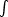g1/2R. Sakharov's arguments make use of the fact that the vacuum fields have inertia (since they are assumed to carry the usual energy-momentum). So, derived inertia comes prior to induced gravity a-la Sakharov. Mechanisms proposed in the literature to produce inertia from vacuum effects (as in refs.  ) also presuppose inertia of the vacuum fields, and can thus not serve as primary mechanisms for inertia.

For the vacuum to be an agent for inertia it is necessary, in the first place, that a non-inertial observer be able to perceive enough details of its motion in the vacuum. The Lorentz invariance built into our theories leads to a vacuum that is, perforce, Lorentz invariant, so uniform motion cannot be detected through it. It is well known, however, that non-inertial motion raises from the vacuum a specter that can be sensed by the observer in different ways . This phenomenon has so far been studied for only a limited class of simple motions. For example, for an observer on a collinear trajectory of constant-acceleration, a, (hyperbolic motion) this avatar of the vacuum is the Unruh radiation: a thermal bath the observer finds itself immersed in, of temperature T = a / 2(= 1, c = 1, k = 1)  . Circular, highly relativistic motions have been discussed, e.g. in refs.  -  where it is found that a single parameter, a =2 v2 / r2 / r, still determines the spectrum of the incarnation of the vacuum (is the Lorentz factor); this is quasi-thermal with effective temperature T =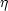a / 2, whereis of order unity and depends somewhat on the frequency. For general motions, hardly anything is known about the radiation. It is clear that the effect must be a nonlocal functional of the whole trajectory, because the relevant wavelengths and frequencies of the radiation may be of the order of scale lengths and frequencies, respectively, that characterize the motion. (For stationary motions, such as the two described above, all points are equivalent, so the Unruh-like radiation appears to depend only on "local" properties. However, the non-local information on the stationarity of the trajectory enters strongly.)

While the Unruh-like radiation may well serve as a marker for non-inertial motions it is still difficult to implicate it directly in the generation of inertia: 1. It is not clear that it carries all the information on the motion needed to produce inertia. For example, even for hyperbolic motion, can the direction of its acceleration be told by the accelerated observer (it should be remembered that the radiation is characterized by more than just its spectrum. For example, a finite size observer can compare the radiation in its different parts.) 2. If inertia is local-as it is to a very good approximation in the non-MOND regime-it has to adjust instantaneously to the state of motion. The latter may change however on time scales that are short compared with the typical period of the Unruh-like radiation. In the MOND regime there is no experimental indication that inertia is local; on the contrary, as mentioned before, theoretical arguments point to nonlocal MOND inertia.

How does MOND fit into this, and, in particular, how can the connection with cosmology be made? When the acceleration of a constant-a observer becomes smaller than a0, the typical frequency of its Unruh radiation becomes smaller than the expansion rate of the Universe, the Unruh wavelength becomes larger than the Hubble distance, etc.  . We expect then some break in the response of the vacuum when we cross the a0 barrier. What is the Unruh radiation seen by a non-inertial observer in a nontrivial universe? We know that even inertial observers in a nontrivial universe find themselves immersed in radiation arising from the distortion of the vacuum. The simplest and best-studied case is that of a de Sitter universe in which all inertial observers see a thermal spectrum with a temperature T= (/ 3)1/3 / 2, whereis the cosmological constant characterizing the de Sitter cosmology. It was shown in refs.   that an observer on an hyperbolic trajectory, in a de Sitter universe, also sees thermal radiation, but with a temperature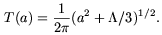(5)

If inertia is what drives a non-inertial body back to (some nearby) inertial state, striving to annul the vacuum radiation-here, for hyperbolic motion, to drive T back to T- then T - Tis a relevant quantity. (With cosmology fixed, the best that inertia can do is drive T to T; in the cosmological context it also strives to drive Tto zero.) We can write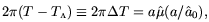(6)

with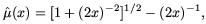(7)

and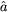0 = 2(/ 3)1/2. The quantityT behaves in just the manner required from MOND inertia  [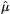(x << 1)x,(x >> 1)1 - (2x)-1] with a0 =0 naturally identified with a cosmological acceleration parameter. (This need not be the effective form of µ for trajectories other than hyperbolic; in mondified inertia there is no µ in the theory itself, and a different form of µ may apply, for instance, to circular orbits  .) While this observation is interesting and suggestive, I cannot tell whether it is germane to MOND, because it is not backed by a concrete mechanism for inertia, and because I cannot generalize the observation to more general motions.

In de Sitter space-time the expansion rate, the space-time curvature, and the cosmological constant are one and the same. These parameters differ from each other in a general Friedmanian universe, and so the above lesson learnt for the de Sitter case does not tell us which of the cosmological acceleration parameters is to be identified with a0 in the real universe.

Recall that, in MOND, inertia vanishes in the limit a0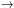. In the above picture this qualitative tenet of MOND is effected because the limit corresponds to, or H0, etc.; so, the Gibbons-Hawking-like radiation due to cosmology swamps the thermal effects due to non-inertial motion: the difference between inertial and non-inertial observers is effaced in this limit.

Acknowledgments

I thank my friends Jacob Bekenstein and Bob Sanders for many helpful comments and discussions from which I have benefited over the years.